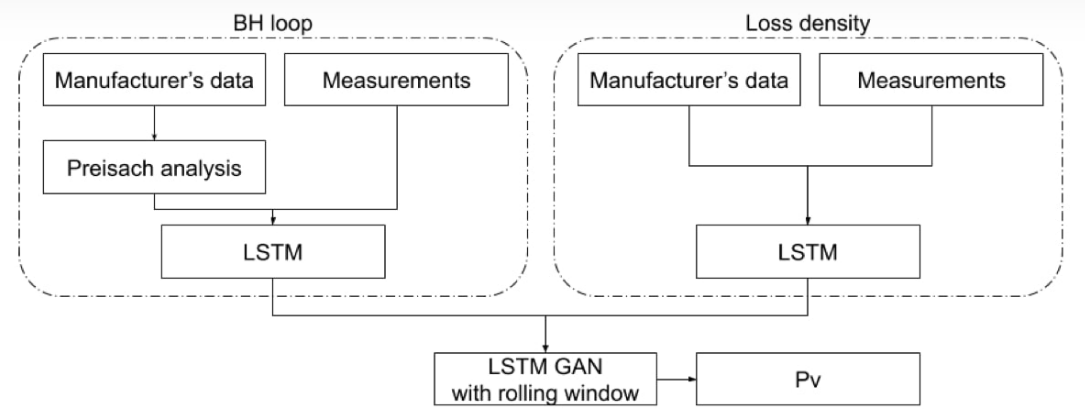# Artificial intelligence for two dependent dimensions

At Frenetic, a couple of years ago we started an AI model to calculate the core losses of magnetics, may have temperature or frequency as a fixed parameter. The temperature or frequency may be static as a parameter. Two cases with sweeps of solutions in two different variables (2 dimensions, 1 is static and the other is traversed).

The constraints subject to the measurement experiments were:

Toroidal inductors,

Turn ratios of 1, 5, 10

Measurements obtained with BS&T

Frequency sweep 1/30kHz

Temperature sweep 25ºC/150ºC

The algorithm used was two GANs + LSTM, one for density losses and the second for hysteresis losses.

On the one hand, the BH loop LSTM is trained by using the unfolded loop as a time series. As a recurrent neural network, it learns from previous states, so the interpretation as a time series mesh speeds up its training. On the other hand, the power loss density LSTM is trained from the error between the measured data and the predictions of the first LSTM loops. The power is calculated through the magnetic energy integral.

(attached image)

The LSTM GAN has a Siamese learning:

1º Specific power loss as a function of maximum flux density with frequency as a parameter.

2º Specific power loss for various combinations of frequency/flux density as a function of the temperature.

Which we can obtain Pv with different parameters.

https://frenetic.ai/application-note/method-for-accurately-predicting-core-losses-using-deep-learning-2

Complete paper:

https://ieeexplore.ieee.org/document/9178051

We found that this architecture was useful for predictions with continuous and representative input variables such as time series (the waveform signals). In this way we could relate each instant “t” of the waveform with the state at “t” of the core and be able to take the precision we have in the calculation of losses with sinusoidal waves to other types of waves, triangular, square... Taking into account the noise and anomalies that these could have.

What do you think about the architecture and the working pipeline?

Do you think that with a neuroevolution neural network, having a recurrent learning philosophy, similar results could be obtained?Edited byon 9 months before
##### Replies